### python云计算,云计算实战系列二十二(Python编程II)

print(“hello,world”)

python内部执行过程如下：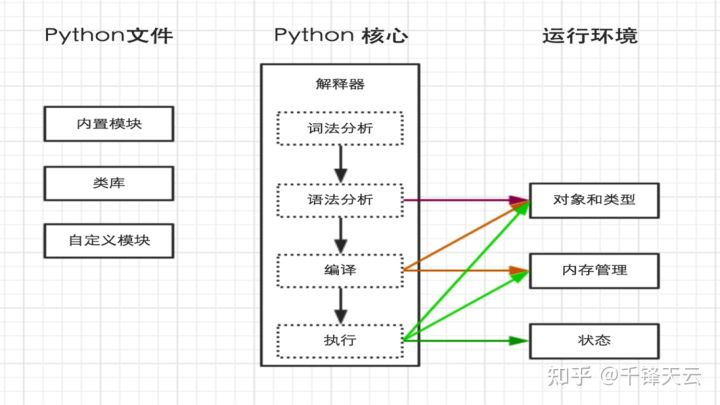#!/usr/bin/env python3

print(“hello,world”)

ps：执行前需给予 hello.py 执行权限，chmod 755 hello.py

#!/usr/bin/env python3

print(“hello,世界”)

python解释器在加载 .py 文件中的代码时，会对内容进行编码(默认ascill)

ASCII(American Standard Code for Information Interchange，美国标准信息交换代码)是基于拉丁字母的一套电脑编码系统，主要用于显示现代英语和其他西欧语言，其最多只能用 8 位来表示(一个字节)，即：2**8 = 256，所以，ASCII码最多只能表示 256 个符号。

Unicode(统一码、万国码、单一码)是一种在计算机上使用的字符编码。Unicode 是为了解决传统的字符编码方案的局限而产生的，它为每种语言中的每个字符设定了统一并且唯一的二进制编码，规定虽有的字符和符号最少由 16 位来表示(2个字节)，即：2 **16 = 65536，

UTF-8，是对Unicode编码的压缩和优化，他不再使用最少使用2个字节，而是将所有的字符和符号进行分类：ascii码中的内容用1个字节保存、欧洲的字符用2个字节保存，东亚的字符用3个字节保存…

Shell 注释方法：单行注释： # 被注释内容多行注释 :<

Python 注释方法单行注释：# 被注释内容多行注释：””” 被注释内容 “””

Python有大量的模块，从而使得开发Python程序非常简洁。类库有包括三中：

Python内部提供的模块业内开源的模块程序员自己开发的模块(温馨提示，python的模块可以是c语言等)

Python内部提供一个 sys 的模块，其中的 sys.argv 用来捕获执行执行python脚本时传入的参数

#!/usr/bin/env python

# -*- coding: utf-8 -*-

import sys

print(sys.argv)

https://www.jianshu.com/p/f0c6130f7b13

ps：代码经过编译可以产生字节码；字节码通过反编译也可以得到代码。

#!/usr/bin/env python

# -*- coding: utf-8 -*-

# 将用户输入的内容赋值给 name 变量

name = input(“请输入用户名：”)

# 打印输入的内容

print(name)

#!/usr/bin/env python

# -*- coding: utf-8 -*-

import getpass

# 将用户输入的内容赋值给 name 变量

pwd = getpass.getpass(“请输入密码：”)

# 打印输入的内容

print(pwd)

1、变量的声明

python语言是动态语言

a = 1

a = ‘千锋’

2、变量命名规则

3、变量命名潜规则

>>> import keyword

>>> keyword.kwlist

[‘False’, ‘None’, ‘True’, ‘and’, ‘as’, ‘assert’, ‘break’, ‘class’, ‘continue’, ‘def’, ‘del’, ‘elif’, ‘else’, ‘except’, ‘finally’, ‘for’, ‘from’, ‘global’, ‘if’, ‘import’, ‘in’, ‘is’, ‘lambda’, ‘nonlocal’, ‘not’, ‘or’, ‘pass’, ‘raise’, ‘return’, ‘try’, ‘while’, ‘with’, ‘yield’]

> 上面打印出来的数据在 Python 中被称为列表， 列表是 Python中一种数据结构。关于数据结构，后面的章节会详细说明。

>列表的都是 Python3 中的关键字。

#可以使用一下方法验证，返回 True 就是 Python 的关键字

>>> keyword.iskeyword(‘del’)

True

>>>

4、变量赋值

python 中使用等号 = 对变量进行赋值,。

n = 5

5、Python 中的变量是什么

s = ‘hello’

hello 这个字符串对象会在内存中先被创建，之后再把变量名 s 分配给这个对象。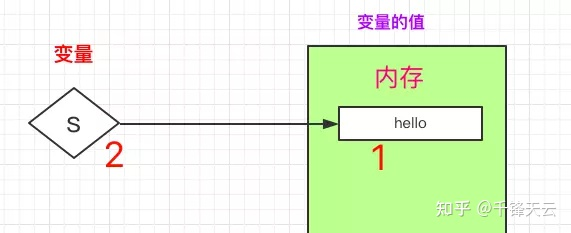a = 1

b = a

a = 2

print(b) # b 会是 ?

a = 1 时如下图：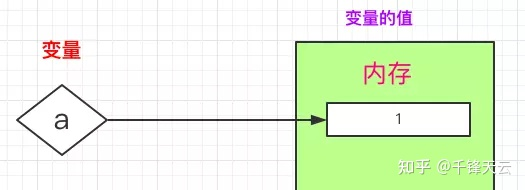b = a 时如下图: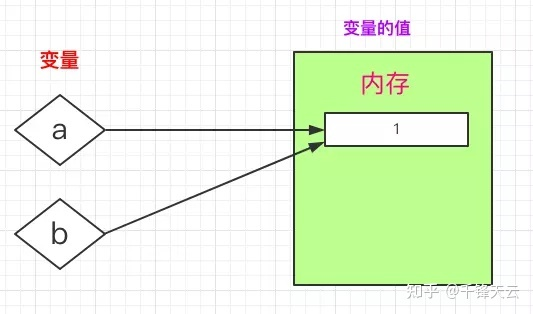a = 2 时如下图: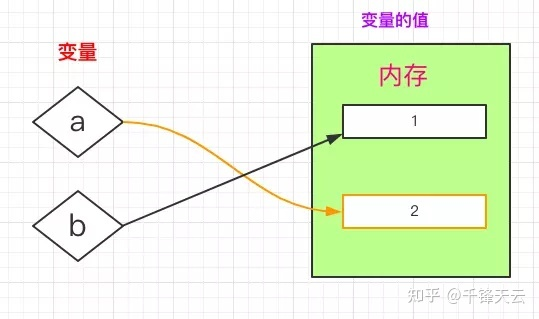6、变量的多元赋值

In : x, y, z = 1, 2, 3

In : x

Out: 1

In : y

Out: 2

In : z

Out: 3

In : a, b, c = ‘abc’

In : a

Out: ‘a’

In : b

Out: ‘b’

In : c

Out: ‘c’

7、Python 中对象(变量的值)都有三个特性

# 唯一标识，是对象在内存中的整数表示形式，在 CPython 中可以理解为# 内存地址

# 可以用 id 这个函数查看

id(10) # 直接给一个对象

id(n) # 给你变量名

# 类型， 对象都有不同的类型，用 type 这个函数查看

type(10)

type(n)

# 值，对象本身

10

1、算数运算

#!/usr/bin/python3

a = 21

b = 10

c = 0

c = a + b

print (“c 的值为：”, c)

c = a – b

print (“c 的值为：”, c)

c = a * b

print (“c 的值为：”, c)

c = a / b

print (“c 的值为：”, c)

c = a % b

print (“c 的值为：”, c)

# 修改变量 a 、b 、c

a = 2

b = 3

c = a**b

print (“c 的值为：”, c)

a = 10

b = 5

c = a//b

print (“c 的值为：”, c)

c 的值为： 31

c 的值为： 11

c 的值为： 210

c 的值为： 2.1

c 的值为： 1

c 的值为： 8

c 的值为： 2

2、比较运算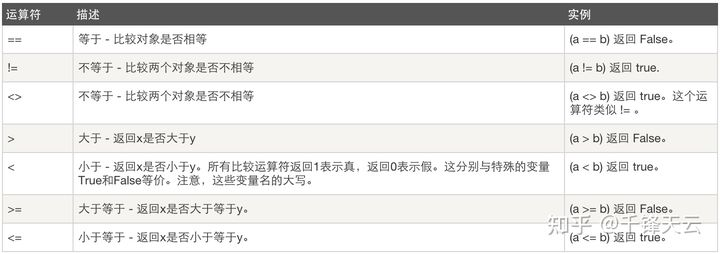3、赋值运算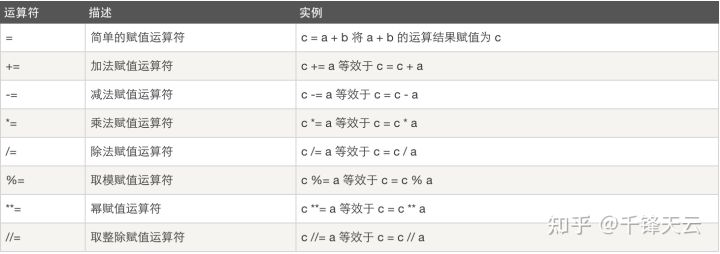#!/usr/bin/python3

a = 21

b = 10

c = 0

if ( a == b ):

print (“a 等于 b”)

else:

print (“a 不等于 b”)

if ( a != b ):

print (“a 不等于 b”)

else:

print (“a 等于 b”)

if ( a < b ):

print (“a 小于 b”)

else:

print (“a 大于等于 b”)

if ( a > b ):

print (“a 大于 b”)

else:

print (“小于等于 b”)

# 修改变量 a 和 b 的值

a = 5;

b = 20;

if ( a <= b ):

print (“a 小于等于 b”)

else:

print (“a 大于 b”)

if ( b >= a ):

print (“b 大于等于 a”)

else:

print (“b 小于 a”)

1 – a 不等于 b

2 – a 不等于 b

3 – a 大于等于 b

4 – a 大于 b

5 – a 小于等于 b

6 – b 大于等于 a

4、逻辑运算

#!/usr/bin/python3

a = 10

b = 20

if ( a and b ):

print (“变量 a 和 b 都为 true”)

else:

print (“变量 a 和 b 有一个不为 true”)

if ( a or b ):

print (“变量 a 和 b 都为 true，或其中一个变量为 true”)

else:

print (“变量 a 和 b 都不为 true”)

# 修改变量 a 的值

a = 0

if ( a and b ):

print (“变量 a 和 b 都为 true”)

else:

print (“变量 a 和 b 有一个不为 true”)

if ( a or b ):

print (“变量 a 和 b 都为 true，或其中一个变量为 true”)

else:

print (“变量 a 和 b 都不为 true”)

if not( a and b ):

print (“变量 a 和 b 都为 false，或其中一个变量为 false”)

else:

print (“变量 a 和 b 都为 true”)

1 – 变量 a 和 b 都为 true

2 – 变量 a 和 b 都为 true，或其中一个变量为 true

3 – 变量 a 和 b 有一个不为 true

4 – 变量 a 和 b 都为 true，或其中一个变量为 true

5 – 变量 a 和 b 都为 false，或其中一个变量为 false

5、成员运算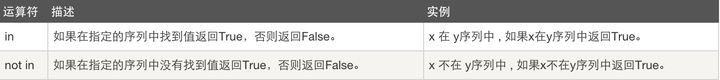#!/usr/bin/python3

a = 10

b = 20

list = [1, 2, 3, 4, 5 ];

if ( a in list ):

print (“变量 a 在给定的列表中 list 中”)

else:

print (“变量 a 不在给定的列表中 list 中”)

if ( b not in list ):

print (“变量 b 不在给定的列表中 list 中”)

else:

print (“变量 b 在给定的列表中 list 中”)

# 修改变量 a 的值

a = 2

if ( a in list ):

print (“变量 a 在给定的列表中 list 中”)

else:

print (“变量 a 不在给定的列表中 list 中”)

1 – 变量 a 不在给定的列表中 list 中

2 – 变量 b 不在给定的列表中 list 中

3 – 变量 a 在给定的列表中 list 中

1、判断

#if 条件语句: # 注意这里必须以英文的冒号结束

# 条件语句为真时，执行的语句

n = input(“输入数字>>:”)

n = int(n) # input 接收到的数据，都是字符串类型

if n == 5:

print(‘相等’)

n = input(“输入数字>>:”)

n = int(n)

if n == 5:

print(‘相等’)

else: # else 后边必须有英文的冒号

print(‘No’)

#_*_ coding:utf-8 _*_

n = input(“输入数字>>:”)

# 必须输入数字来测试

if not n: # 如果为空

print(“空值”) # 输出 空值

else: #否则

n = int(n) # n 转化为整型

if n == 5: # 如果等于5

print(‘ok’) # 输出ok

elif n > 5: # 如果 大于5

print(‘大了’) # 输出大了

else: # 否则

print(‘小了’) #输出小了

2、嵌套

n = input(“输入数字>>:”)

if n.isdigit():

f_n = int(n)

if f_n == 5:

print(‘ok’)

elif f_n > 5:

print(‘大了’)

else:

print(‘小了’)

else:

print(‘请输入数字’)

3、循环

while True:

n = input(“输入数字>>:”)

n = int(n)

if n == 5:

print(‘相等’)

break

elif n > 5:

print(‘大了’)

else:

print(‘小了’)

4、迭代

for 循环(英语：for loop)

for i in ‘hello world’:

print(i)

range(n)

for i in range(5):

print(i)

break 和 continue

for i in range(0, 10):

print(i)

if i < 3:

inp_num = int(input(“>>:”).strip())

# inp_num = int(inp_num)

if inp_num == 15:

print(‘You get it’)

break

elif inp_num > 15:

print(“高了”)

else:

print(“低了”)

else:

print(“Game over”)

break

for i in range(2, 10, 2):

print(“循环到”, i)

if i == 4:

continue

print(i)

if i == 6:

break

range(开始索引号:结束索引号:步长) 其道理和切片一样

5、扩展知识

for … else

•1. 当 for 循环体正常执行完毕时，再执行一下 else 后面的代码

•2. 当 执行了 break 后，就不再执行 else 后面的代码了

for i in range(2):

if i == 10:

print(‘get it’)

n = i

break

else:

print(“Nothing”)返回搜狐，查看更多